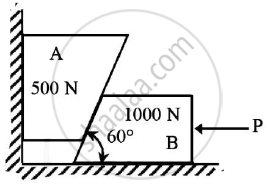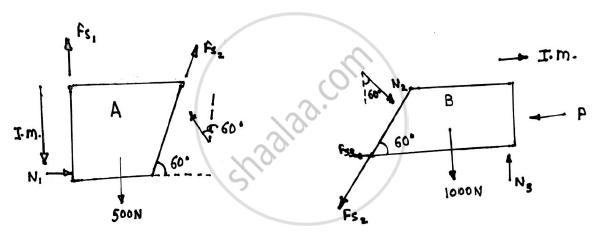Advertisement Remove all ads

# Two blocks A and B are resting against the wall and floor as shown in the figure.Find the minimum value of P that will hold the system in equilibrium. - Engineering Mechanics

Answer in Brief

Two blocks A and B are resting against the wall and floor as shown in the figure.Find the minimum value of P that will hold the system in equilibrium. Take μ=0.25 at the floor,μ=0.3 at the wall and μ=0.2 between the blocks.Given : μ=0.25 at floor
μ=0.2 between blocks
To find : Minimum value of force P

Advertisement Remove all ads

#### SolutionThe impending motion of block A is to move down and that of block B is to move towards left
FS1=μ1N1=0.3N1
FS22N2=0.2N2
FS33N3=0.25N3 ……………..(1)
Block A is under equilibrium
Applying conditions of equilibrium

ΣFY=0
-500+FS1+FS2sin60+N2cos60=0
0.3N1+0.6732N2=500 ………….(2)

Similarly, ΣFX=0

N1+FS2cos60-N2sin60=0

N1+0.2N2 x 0.5 – N2 x 0.866 = 0 (From 1)

N1-0.766N2=0 ……….(3)

Solving (2) and (3)

N1=424.1417 N

N2=553.71 N

Applying conditions of equilibrium on block B

ΣFY=0
-1000+N3-FS2sin60-N2 cos60=0
N3-0.6732N2=1000
N3=1372.7576 N
ΣFX=0
-P-FS3-FS2cos60+N2sin60=0
-0.25N3-0.2N2 x 0.5 +N2 x 0.866 = P
P=80.9525 N
The minimum value of force P that will hold the system in equilibrium is 80.9525 N

Concept: Condition of equilibrium for concurrent forces
Is there an error in this question or solution?
Advertisement Remove all ads

#### APPEARS IN

Advertisement Remove all ads
Advertisement Remove all ads
Share
Notifications

View all notifications

Forgot password?